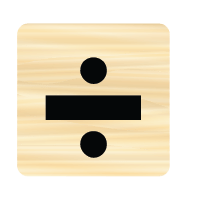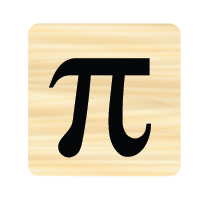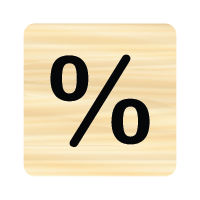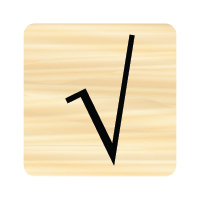# Symbols Picture Quiz

Can you name these symbols?

 1a) at b) infinity c) percent at infinity percent 2a) square root b) multiplied by c) divided by square root multiplied by divided by 3a) multiplied by b) hash tag or pound sign c) approximately multiplied by hash tag or pound sign approximately 4a) pi b) infinity c) at pi infinity at 5a) percent b) square root c) divided by percent square root divided by 6a) hash tag or pound sign b) multiplied by c) divided by hash tag or pound sign multiplied by divided by 7a) pi b) and c) at pi and at 8a) at b) and c) about at and about 9a) hash tag or pound sign b) multiplied by c) percent hash tag or pound sign multiplied by percent 10a) infinity b) divided by c) square root infinity divided by square root